## Thursday, February 18, 2016

### Small update to default arguments of `reroot`

I just updated the phytools function `reroot` so that the default `position` along the edge at which to re-root the tree is at the `node.number` node supplied by the user. Previously, the function would not run when `position` was not supplied.

E.g.:

``````library(phytools)
plotTree(tree,offset=0.8)
nodelabels()
tiplabels()
``````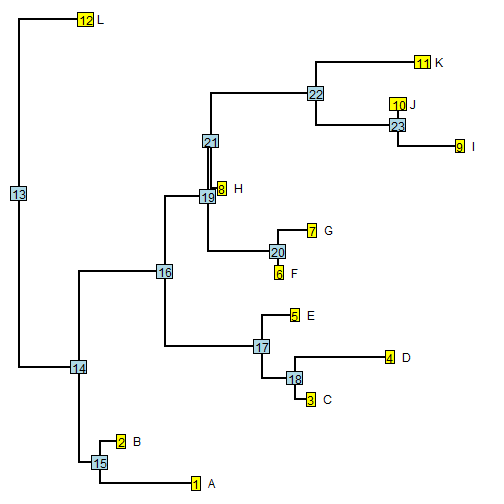Now, let's perform operations on this original tree:

``````## reroot 0.1 along edge leading to node 22
t_22<-reroot(tree,node.number=22,position=0.1)
plotTree(t_22)
``````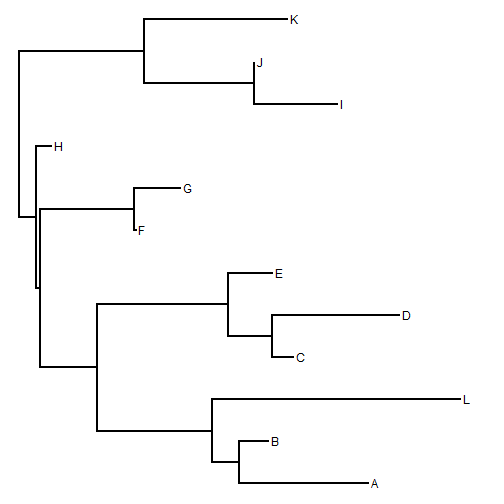``````## reroot halfway along edge leading to tip "D"
node<-which(tree\$tip.label=="D")
position<-0.5*tree\$edge.length[which(tree\$edge[,2]==node)]
t_D<-reroot(tree,node,position)
plotTree(t_D)
``````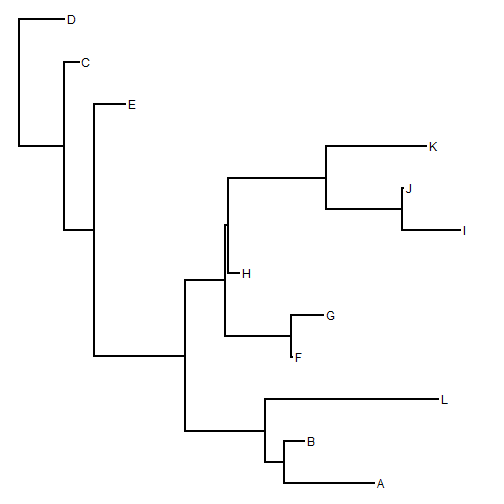``````## reroot at node 16
t_16<-reroot(tree,16)
plotTree(t_16)
``````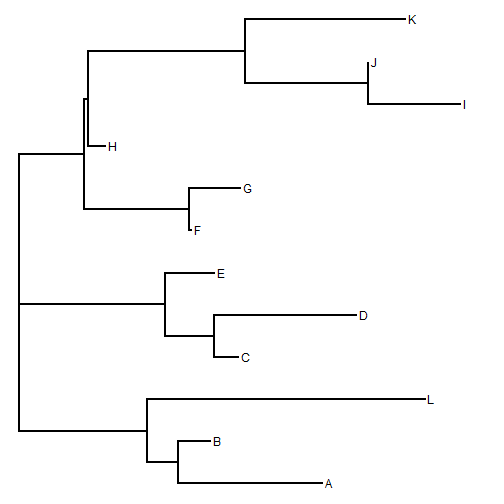``````## reroot at tip "A"
plotTree(t_A<-reroot(tree,which(tree\$tip.label=="A")))
``````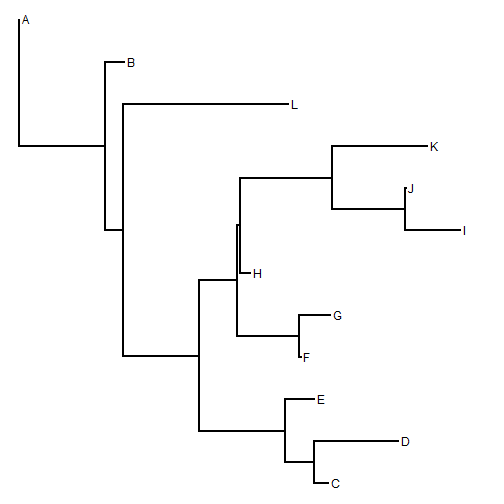Note that in this function the argument `position` is the distance from the rootward end of the edge leading to node `node.number` at which the tree should be re-rooted. Also, though it is hard to demonstrate here, the function now has an `interactive=TRUE` mode (described here).